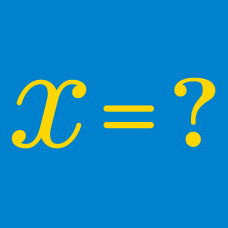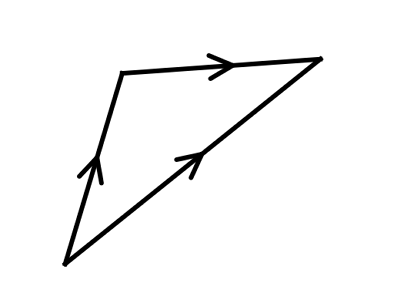Algebra

Algebra Warmups: Level 3 Challenges

What is the value of

$\large \sqrt{1\text{\%}}\, ?$

$\frac{100001+100003+100005+\cdots+199999}{1+3+5+7+\cdots+99999} = \, ?$Over all real numbers $x$, find the minimum value of $\sqrt{(x+6)^2+25} + \sqrt{(x-6)^2+121}$.

$\large (x+3)(x+4)(x+6)(x+7) = 1120$

Find the sum of all reals $x$ satisfying the equation above.

$\large \sqrt{-2}\times\sqrt{-3}=\, ?$

In this problem, the square root is a function from the complex numbers to the complex numbers.

×